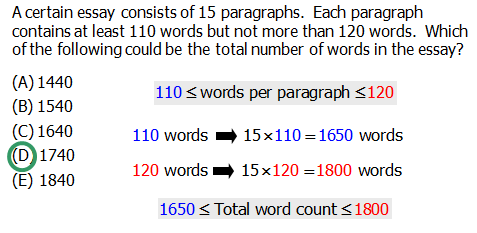# Doubling and Halving Trick

Learn one of the handiest tricks for math without a calculator!

Without a calculator, what is

Q1. 35 \times 12?

Q2. 150 \times 36?

Q3. 125 \times 84?

Q4. A certain essay consists of 15 paragraphs. Each paragraph contains at least 110 words but not more than 120 words. Which of the following could be the total number of words in the essay?

A. 1440

B. 1540

C. 1640

D. 1740

E. 1840

On the QUANT, you don't get a calculator. With the doubling and halving trick, all of these become much easier.

Every positive integer has a unique prime factorization — that is to say, there is a unique way to express each whole number as a product of prime factors. Therefore, whenever we multiply to positive integers, we can think of this the product of the prime factors of one times the product of the prime factors of the other — two big collections of factors being multiplied together. Furthermore, the associative law the commutative law tell us we can multiply in any order — we could even swap around factors from one number to the other, and the overall result of the multiplication would not change.

## Doubling and halving

Suppose one factor ends in 5, and suppose the other factor is even. In this case, we know the even factor must be divisible by 2, so we can easily remove a factor of 2 from that one (thereby "halving" it) and multiply the multiple of 5 by 2 (thereby "doubling" it), which will make that one a multiple of ten. In this process, both numbers become simpler, and the multiplication often becomes something you could easily do in your head.

For example, consider the multiplication 15 \times 16. At first glance, that looks not-fun without a calculator. Now, we will perform "doubling and halving." Remove a factor of 2 from 16, so 16 becomes 8 — it "halves." Give that spare factor of 2 to 15 — multiply 15 by 2 to get 30. Therefore, 15 \times 16 = 30 \times 8 = 240. After using the doubling-and-halving trick, the problem just becomes one-digit multiplication, with an extra zero along for the ride.

In the case of 25 \times 44, we can do doubling-and-halving once — 44 becomes 22 and 25 becomes 50 — to get 25 \times 44 = 50 \times 22. That's better, but we can do doubling-and-halving again — 22 becomes 11 and 50 becomes 100 — so that 25 \times 44 = 50 \times 22 = 100 \times 11 = 1100.

Take another look at the problems at the top, and see if you can simplify them with the doubling-and-halving trick before reading the solutions below.

## Solutions

Q1. Halve 12, so it becomes 6. Double 35, so it becomes 70.

12 \times 35 = 6 \times 70 = \bold{420}.

Q2. Halve 36, so it becomes 18. Double 150, so it becomes 300.

150 \times 36 = 300 \times 18 = \bold{5400}.

Q3. Halve 84, so it comes 42. Double 125, so it becomes 250. 125 \times 84 = 250 \times 42. Now, perform the trick again. Halve 42, so it becomes 21. Double 250, so it becomes 500.

125 \times 84 = 250 \times 42 = 500 \times 21.

Now, notice that 21 = 20 + 1,

so 500 \times 21 = 500 \times (20 + 1) = 500 \times 20 + 500

Apply doubling & halving one more time, 500 to 1000, and 20 to 10:

500 \times 21 = 500 \times 20 + 500 = 1000 \times 10 + 500 = 10000 + 500 = \bold{10500}.

Q4.No calculator, no problem!